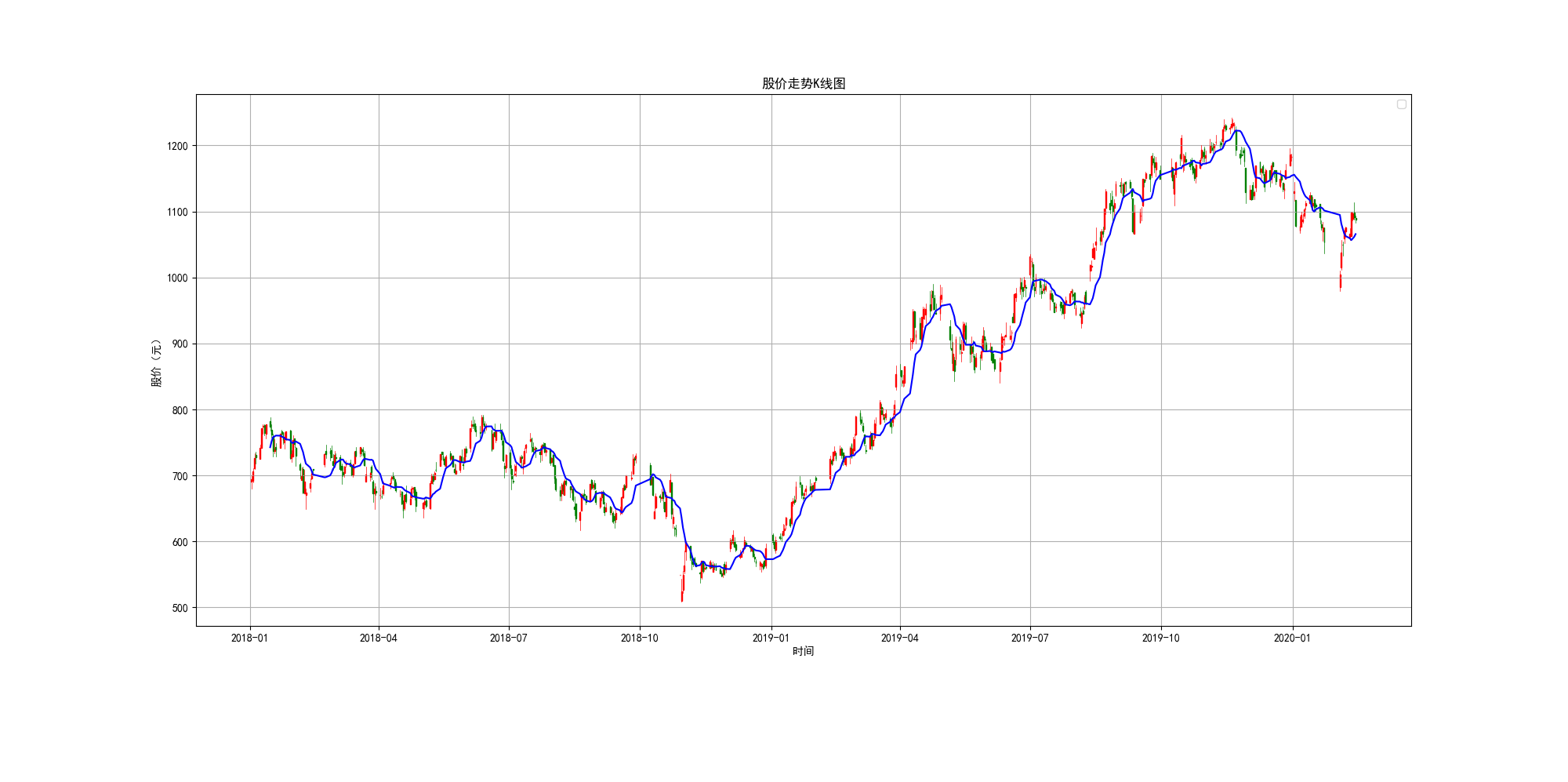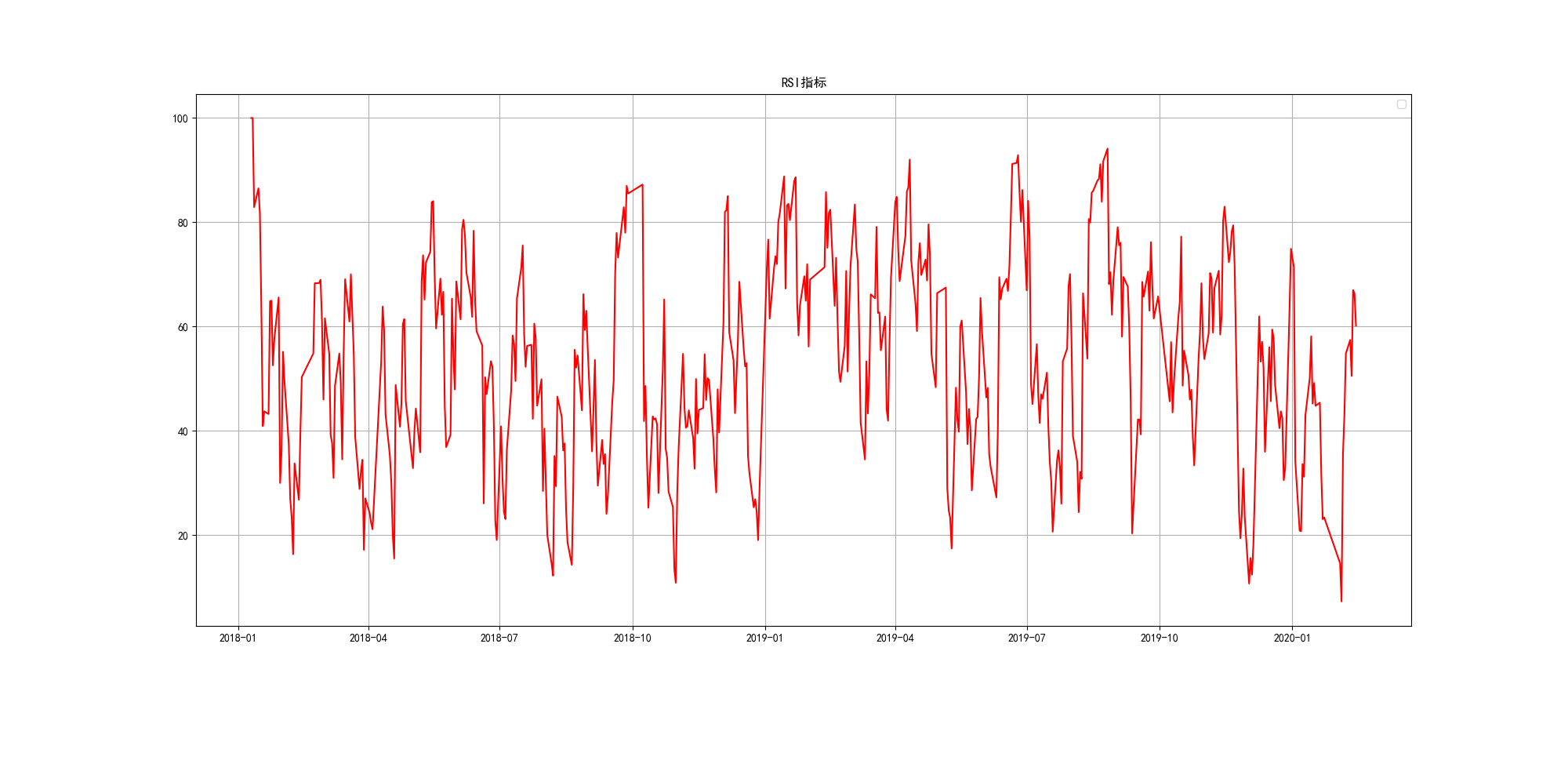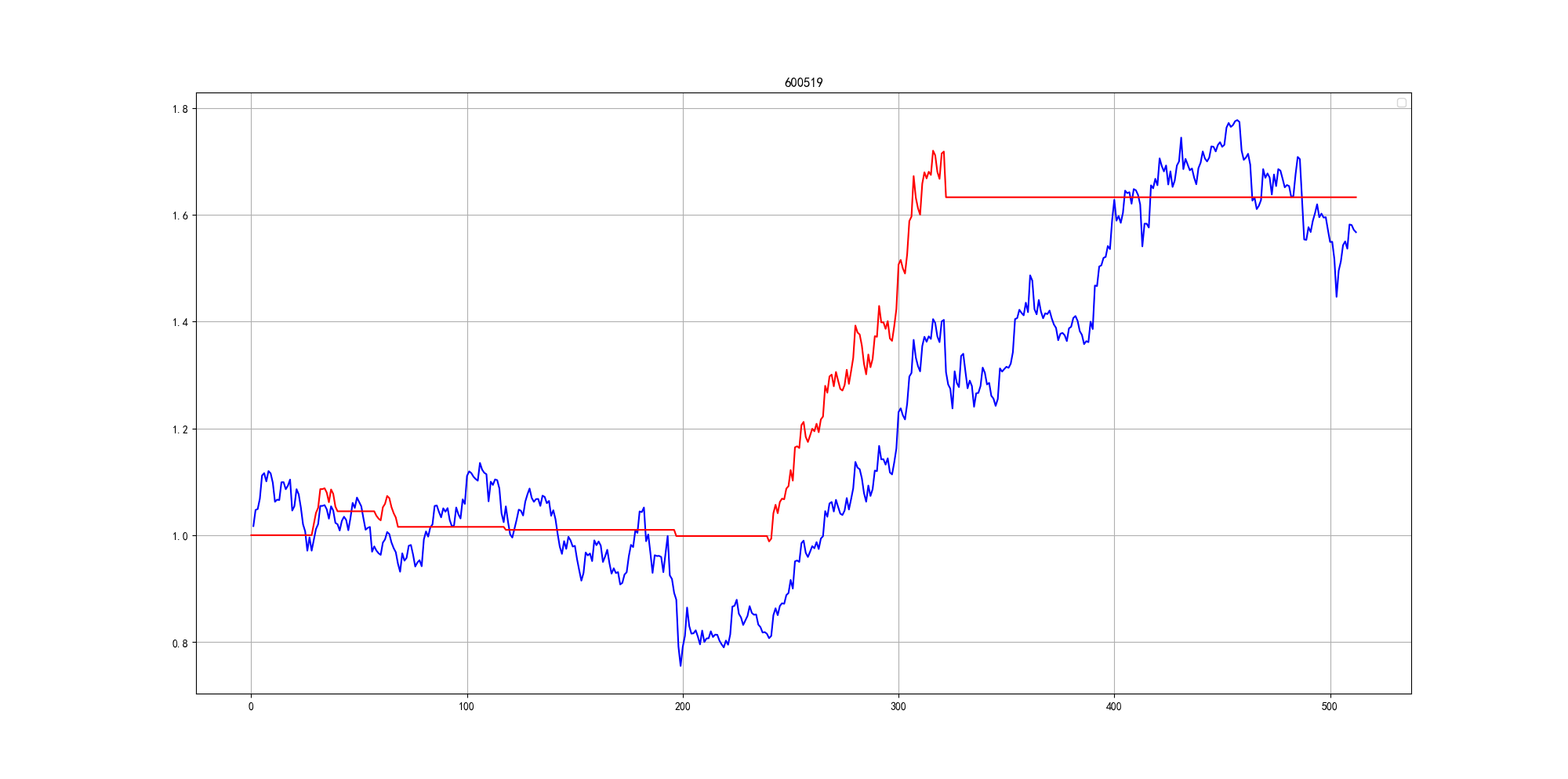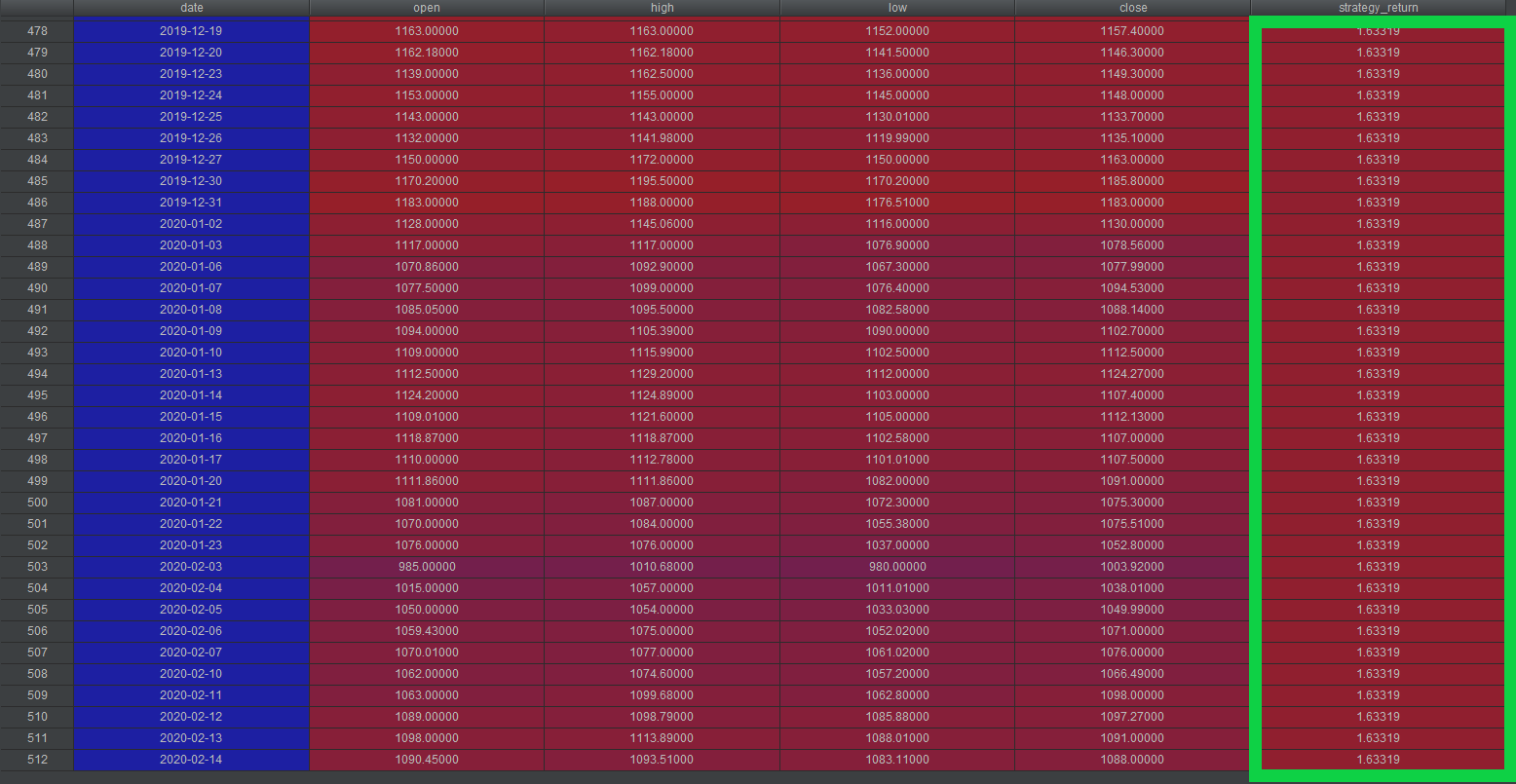• 问：如何使用Python分析大数据毫不夸张地说，大数据已经成为任何商业交流中不可或缺一部分。桌面和移动搜索向全世界营销人员和公司以空前规模提供着数据，并且随着物联网到来，大量用以消费数据还会呈指数...
问：如何使用Python分析大数据毫不夸张地说，大数据已经成为任何商业交流中不可或缺的一部分。桌面和移动搜索向全世界的营销人员和公司以空前的规模提供着数据，并且随着物联网的到来，大量用以消费的数据还会呈指数级增长。这种消费数据对于想要更好地定位目标客户、弄懂人们怎样使用他们的产品或服务，并且通过收集信息来提高利润的公司来说无疑是个金矿。筛查数据并找到企业真正可以使用的结果的角色落到了软件开发者、数据科学家和统计学家身上。现在有很多工具辅助大数据分析，但最受欢迎的就是Python。为什么选择Python?Python最大的优点就是简单易用。这个语言有着直观的语法并且还是个强大的多用途语言。这一点在大数据分析环境中很重要，并且许多企业内部已经在使用Python了，比如Google，YouTube，迪士尼，和索尼梦工厂。还有，Python是开源的，并且有很多用于数据科学的类库。所以，大数据市场急需Python开发者，不是Python开发者的专家也可以以相当块速度学习这门语言，从而最大化用在分析数据上的时间，最小化学习这门语言的时间。用Python进行数据分析之前，你需要从Continuum.io下载Anaconda。这个包有着在Python中研究数据科学时你可能需要的一切东西。它的缺点是下载和更新都是以一个单元进行的，所以更新单个库很耗时。但这很值得，毕竟它给了你所需的所有工具，所以你不需要纠结。现在，如果你真的要用Python进行大数据分析的话，毫无疑问你需要成为一个Python开发者。这并不意味着你需要成为这门语言的大师，但你需要了解Python的语法，理解正则表达式，知道什么是元组、字符串、字典、字典推导式、列表和列表推导式——这只是开始。各种类库当你掌握了Python的基本知识点后，你需要了解它的有关数据科学的类库是怎样工作的以及哪些是你需要的。其中的要点包括NumPy，一个提供高级数学运算功能的基础类库，SciPy，一个专注于工具和算法的可靠类库，Sci-kit-learn，面向机器学习，还有Pandas，一套提供操作DataFrame功能的工具。除了类库之外，你也有必要知道Python是没有公认的最好的集成开发环境(IDE)的，R语言也一样。所以说，你需要亲手试试不同的IDE再看看哪个更能满足你的要求。开始时建议使用IPython Notebook，Rodeo和Spyder。和各种各样的IDE一样，Python也提供各种各样的数据可视化库，比如说Pygal，Bokeh和Seaborn。这些数据可视化工具中最必不可少的就是Matplotlib，一个简单且有效的数值绘图类库。所有的这些库都包括在了Anaconda里面，所以下载了之后，你就可以研究一下看看哪些工具组合更能满足你的需要。用Python进行数据分析时你会犯很多错误，所以得小心一点。一旦你熟悉了安装设置和每种工具后，你会发现Python是目前市面上用于大数据分析的最棒的平台之一。希望能帮到你！
展开全文• 最近在研究一些股票的东西，想批量获取所有沪深股票基本面的数据，然后就上网搜索到一个非常好的股票python接口包Tushare，而且使用方法超级简单，很适合我这种python初学者菜鸟。1.首先需要先下载这个接口包，我是...
最近在研究一些股票的东西，想批量获取所有沪深股票基本面的数据，然后就上网搜索到一个非常好的股票python接口包Tushare，而且使用方法超级简单，很适合我这种python初学者菜鸟。1.首先需要先下载这个接口包，我是使用anaconda3,然后直接打开anaconda promt,然后直接输入pip install tushare2.接着你就可以在spyder里直接导入tushare的包了import pandas as pdimport numpy as npimport osimport tushare as tsos.chdir(r'C:\Users\jianminli\Desktop')#更改路径basic=ts.get_stock_basics()#调用tushre包的函数获取上市公司基本面的数据basic.to_excel("上市公司基本面.xlsx")#把数据读入到excel里代码就几行，超级简单以下还有很多参考的函数#获取2014年第3季度的业绩报表数据ts.get_report_data(2014,3)#获取2014年第3季度的盈利能力数据ts.get_profit_data(2014,3)#获取2014年第3季度的营运能力数据ts.get_operation_data(2014,3)#获取2014年第3季度的成长能力数据ts.get_growth_data(2014,3)#获取2014年第3季度的偿债能力数据ts.get_debtpaying_data(2014,3)#获取2014年第3季度的现金流量数据ts.get_cashflow_data(2014,3)
展开全文• """利用python进行股票技术分析--以茅台为例通过下述策略利用python进行股票技术分析，可以举一反三，并且能够实现复杂技术分析策略，同时可以同python的宏观数据、公司基本面数据、舆情等分析结合起来。...
"""利用python进行股票技术分析--以茅台为例通过下述策略对利用python进行股票技术分析，可以举一反三，并且能够实现复杂的技术分析策略，同时可以同python的宏观数据、公司基本面数据、舆情等分析结合起来。策略原理：在股票下跌过程中，当出现上涨锤子线，显示行情先继续下跌后出现大幅反弹，行情可能由此反转;利用均线识别下跌趋势，以下跌趋势中出现锤子线，同时以RSI出现超卖，三种信号出现作为开仓信号;采用移动止损方式进行止损，止损条件：当天最低价 < max(均价-观察期内一定倍数的标准差，开仓价-开仓时标准差)"""# 1、设置参数变量stock_code='600519' #股票代码start_time='2018-01-01' #开始时间end_time='2020-02-15'   #截止时间body_range=0.05     #实体线波动范围head_length=0.5     #上影线长度tail_length=2       #下影线长度ma_period=9        #均线周期rsi_period=5        #rsi周期rsi_oversell=30     #超卖stoplose_std=2      #止损标准差倍数# 2、抓取股票日线数据并加工处理stock_data=ts.get_k_data(stock_code,start=start_time,end=end_time)stock_data.sort_index(ascending=True,inplace=True)stock_data.reset_index(inplace=True)del stock_data['volume']del stock_data['code']stock_data=pd.DataFrame(stock_data)#计算每天的收益变化stock_data['perday_change']=stock_data['close'].pct_change()#计算指标,当天交易的时候只能用上一天的数据：stock_data['ma']=ta.SMA(np.asarray(stock_data['close']),timeperiod=ma_period)stock_data['rsi']=ta.RSI(np.asarray(stock_data['close']),timeperiod=rsi_period)stock_data['std']=stock_data['close'].rolling(ma_period).std()stock_data['y_ma']=stock_data['ma'].shift(1)stock_data['y_rsi']=stock_data['rsi'].shift(1)stock_data['y_std']=stock_data['std'].shift(1)#计算锤子线形态并判断是否为锤子线:实体线变化范围在一定范围内，下影线要是实体线的一定倍数，上影线要小于下影线一定倍数，当天交易的时候只能用上一天的数据：stock_data['st_body']=abs(stock_data['open']-stock_data['close'])stock_data['s_head']=stock_data['high']-stock_data[['open','close']].max(axis=1)stock_data['x_tail']=stock_data[['open','close']].min(axis=1)-stock_data['low']stock_data['r_body']=np.where(stock_data['st_body']/stock_data['open']stock_data['r_head']=np.where(stock_data['s_head']==0,False,stock_data['s_head']/stock_data['x_tail']stock_data['r_tail']=np.where(stock_data['st_body']==0,True,stock_data['x_tail']/stock_data['st_body']>tail_length)stock_data['chuizi']=stock_data[['r_body','r_head','r_tail']].all(axis=1)stock_data['y_chuizi']=stock_data['chuizi'].shift(1)#3、绘图显示股票的价格、均线、RSI# 对ts获取到的数据转换成candlestick_ohlc()方法可读取的格式data_list = []data_ma=[]for dates,row in stock_data.iterrows():# 将时间转换为数字date_time = datetime.datetime.strptime(row['date'],'%Y-%m-%d')t = date2num(date_time)open,high,low, close= row[['open','high','low','close']]datas = (t,open,high,low,close)data_list.append(datas)    data_ma.append(t)# 创建子图fig, ax = plt.subplots(figsize=(20, 10))fig.subplots_adjust(bottom=0.2)#子图所在区域的边界ax.xaxis_date()# 设置X轴刻度为日期时间plt.xticks()plt.yticks()plt.title("股价走势K线图")plt.xlabel("时间")plt.ylabel("股价(元)")mpf.candlestick_ohlc(ax,data_list,width=0.5,colorup='r',colordown='green')plt.plot(data_ma,stock_data['y_ma'],color='b')plt.legend()plt.grid()fig, ax = plt.subplots(figsize=(20, 10))fig.subplots_adjust(bottom=0.2)#子图所在区域的边界ax.xaxis_date()# 设置X轴刻度为日期时间plt.plot(data_ma,stock_data['y_rsi'],color='r')plt.title('RSI指标')plt.legend()plt.grid()#4、计算交易过程和逻辑编写：只有同时满足均线、RSI和锤子线三者条件才下单，并计算收益和止损flag=0 #flag表示持仓记录，0代表空仓，1代表持仓for i in range(2*ma_period,len(stock_data)):if flag==0:#满足三个条件才开仓，并计算收益if stock_data.loc[i-ma_period,'y_ma']>stock_data.loc[i,'y_ma'] and stock_data.loc[i,'y_rsi']flag=1long_openprice=stock_data.loc[i,'open']long_gap=stock_data.loc[i,'y_std']stock_data.loc[i,'return']=stock_data.loc[i,'close']/stock_data.loc[i,'open']-1stock_data.loc[i,'mark']=100if flag==1:#设置止损价格，达到止损条件平仓long_stoplose=max(stock_data.loc[i,'y_ma']-stoplose_std*stock_data.loc[i,'y_std'],long_openprice-long_gap)if stock_data.loc[i,'low']flag=0stock_data.loc[i,'return']=min(stock_data.loc[i,'open'],long_stoplose)/stock_data.loc[i-1,'close']-1stock_data.loc[i,'mark']=-100else:#持仓过程中计算收益stock_data.loc[i,'return']=stock_data.loc[i,'close']/stock_data.loc[i-1,'close']-1stock_data.loc[i,'mark']=200#5、计算策略收益率并绘图,通过曲线图直观显示收益情况,从图表中显示最终获得收益为1.63倍。stock_data['return'].fillna(0,inplace=True)stock_data['strategy_return']=(stock_data['return']+1).cumprod()stock_data['stock_return']=(stock_data['perday_change']+1).cumprod()fig2 = plt.figure(figsize=(20,10))ax2 = fig2.add_subplot(1,1,1)ax2.plot(stock_data.stock_return,color='b')ax2.plot(stock_data.strategy_return,color='r')plt.title(stock_code)plt.legend()plt.grid()展开全文• 通过下述策略利用python进行股票技术分析，可以举一反三，并且能够实现复杂技术分析策略，同时可以同python的宏观数据、公司基本面数据、舆情等分析结合起来。 策略原理：在股票下跌过程中，当出现上涨锤子线...
“”"
利用python进行股票技术分析–以茅台为例
 通过下述策略对利用python进行股票技术分析，可以举一反三，并且能够实现复杂的技术分析策略，同时可以同python的宏观数据、公司基本面数据、舆情等分析结合起来。
策略原理：在股票下跌过程中，当出现上涨锤子线，显示行情先继续下跌后出现大幅反弹，行情可能由此反转;
利用均线识别下跌趋势，以下跌趋势中出现锤子线，同时以RSI出现超卖，三种信号出现作为开仓信号;
采用移动止损方式进行止损，止损条件：当天最低价 < max(均价-观察期内一定倍数的标准差，开仓价-开仓时标准差）

“”"
1、设置参数变量
stock_code='600519' #股票代码
start_time='2018-01-01' #开始时间
end_time='2020-02-15'   #截止时间
body_range=0.05     #实体线波动范围
tail_length=2       #下影线长度
ma_period=9        #均线周期
rsi_period=5        #rsi周期
rsi_oversell=30     #超卖
stoplose_std=2      #止损标准差倍数

2、抓取股票日线数据并加工处理
stock_data=ts.get_k_data(stock_code,start=start_time,end=end_time)
stock_data.sort_index(ascending=True,inplace=True)
stock_data.reset_index(inplace=True)
del stock_data['volume']
del stock_data['code']
stock_data=pd.DataFrame(stock_data)

#计算每天的收益变化
stock_data['perday_change']=stock_data['close'].pct_change()

#计算指标,当天交易的时候只能用上一天的数据：
stock_data['ma']=ta.SMA(np.asarray(stock_data['close']),timeperiod=ma_period)
stock_data['rsi']=ta.RSI(np.asarray(stock_data['close']),timeperiod=rsi_period)
stock_data['std']=stock_data['close'].rolling(ma_period).std()

stock_data['y_ma']=stock_data['ma'].shift(1)
stock_data['y_rsi']=stock_data['rsi'].shift(1)
stock_data['y_std']=stock_data['std'].shift(1)

#计算锤子线形态并判断是否为锤子线:实体线变化范围在一定范围内，下影线要是实体线的一定倍数，上影线要小于下影线一定倍数，当天交易的时候只能用上一天的数据：
stock_data['st_body']=abs(stock_data['open']-stock_data['close'])
stock_data['x_tail']=stock_data[['open','close']].min(axis=1)-stock_data['low']
stock_data['r_body']=np.where(stock_data['st_body']/stock_data['open']<body_range,True,False)
stock_data['r_tail']=np.where(stock_data['st_body']==0,True,stock_data['x_tail']/stock_data['st_body']>tail_length)
stock_data['y_chuizi']=stock_data['chuizi'].shift(1)

3、绘图显示股票的价格、均线、RSI
对ts获取到的数据转换成candlestick_ohlc()方法可读取的格式
data_list = []
data_ma=[]
for dates,row in stock_data.iterrows():
# 将时间转换为数字
date_time = datetime.datetime.strptime(row['date'],'%Y-%m-%d')
t = date2num(date_time)
open,high,low, close= row[['open','high','low','close']]
datas = (t,open,high,low,close)
data_list.append(datas)
data_ma.append(t)创建子图
fig, ax = plt.subplots(figsize=(20, 10))
ax.xaxis_date()# 设置X轴刻度为日期时间
plt.xticks()
plt.yticks()
plt.title("股价走势K线图")
plt.xlabel("时间")
plt.ylabel("股价（元）")
mpf.candlestick_ohlc(ax,data_list,width=0.5,colorup='r',colordown='green')
plt.plot(data_ma,stock_data['y_ma'],color='b')
plt.legend()
plt.grid()

fig, ax = plt.subplots(figsize=(20, 10))
ax.xaxis_date()# 设置X轴刻度为日期时间
plt.plot(data_ma,stock_data['y_rsi'],color='r')
plt.title('RSI指标')
plt.legend()
plt.grid()4、计算交易过程和逻辑编写：只有同时满足均线、RSI和锤子线三者条件才下单，并计算收益和止损
flag=0 #flag表示持仓记录，0代表空仓，1代表持仓
for i in range(2*ma_period,len(stock_data)):
if flag==0:
#满足三个条件才开仓，并计算收益
if stock_data.loc[i-ma_period,'y_ma']>stock_data.loc[i,'y_ma'] and stock_data.loc[i,'y_rsi']<rsi_oversell and stock_data.loc[i,'y_chuizi']:
flag=1
long_openprice=stock_data.loc[i,'open']
long_gap=stock_data.loc[i,'y_std']
stock_data.loc[i,'return']=stock_data.loc[i,'close']/stock_data.loc[i,'open']-1
stock_data.loc[i,'mark']=100
if flag==1:
#设置止损价格，达到止损条件平仓
long_stoplose=max(stock_data.loc[i,'y_ma']-stoplose_std*stock_data.loc[i,'y_std'],long_openprice-long_gap)
if stock_data.loc[i,'low']<long_stoplose:
flag=0
stock_data.loc[i,'return']=min(stock_data.loc[i,'open'],long_stoplose)/stock_data.loc[i-1,'close']-1
stock_data.loc[i,'mark']=-100
else:
#持仓过程中计算收益
stock_data.loc[i,'return']=stock_data.loc[i,'close']/stock_data.loc[i-1,'close']-1
stock_data.loc[i,'mark']=200

5、计算策略收益率并绘图,通过曲线图直观显示收益情况,从图表中显示最终获得收益为1.63倍。
stock_data['return'].fillna(0,inplace=True)
stock_data['strategy_return']=(stock_data['return']+1).cumprod()
stock_data['stock_return']=(stock_data['perday_change']+1).cumprod()fig2 = plt.figure(figsize=(20,10))
ax2.plot(stock_data.stock_return,color='b')
ax2.plot(stock_data.strategy_return,color='r')
plt.title(stock_code)
plt.legend()
plt.grid()展开全文大数据 算法 数据挖掘 机器学习
• Q1：求比较好用的股票自动交易软件机财智自动交易软件...区间交易策略不对股票未来走势进行分析和预测，也不需要研究股票的基本面、消息面，通过模型同时设定上涨、横盘、下跌这三种情况的应对策略，实现目标股票...
• 所谓“多因子模型”，说白了就是寻找那些对股票收益率最相关影响因素，使用这些因素(因子或指标)来刻画股票收益并进行选股。多因子模型是量化投资领域应用最广泛也是最成熟量化选股模型之一，建立在投资组合、...
• 由于在2月18日之后，大盘产生剧烈波动，在此期间白马股资金反应十分平淡，存在白马股下跌情形，所以想利用tushare筛选2-18日到3-9日这几十天内上涨的股票，并进一步分析其特征。基本思路如下，程序在最后。 1....
• 随着我国证券市场的不断壮大证券及证券投资在社会经济生活中的地位也...分析外还希望这些股票的基本面及市场交易机会进行客观理性地评估传统的基本面分析投资方法主要是通过实地调研阅读公司投资及经营方面的公告...
• 基本分析法着重于一般经济情况以及各个公司的经营管理状况、行业动态等因素进行分析，以此来研究股票的价值，衡量股价的高低。而技术分析则是透过图表或技术指标的记录，研究市场过去及现在的行为反应，以推测未来...# python对股票的基本面进行分析python 订阅